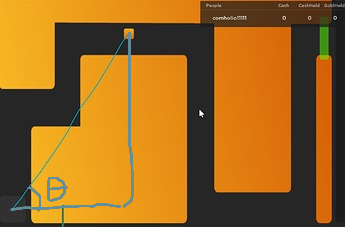# Getting distance of two GUI components and drawing a line to both

I’m working on a minigame and im trying to draw a line to the player and the StartNode but it isnt working how would i fix this? everything is calculated but i want to center the line between both components, size them correctly and rotate them.

Example:

Code:

``````local UserInterfaceTrailService = {}

local DefaultConfig = {
TrailColor = Color3.new(0.250980, 0.474509, 0.341176),
TrailOffset = UDim2.new(0, 0),
}

local UICorner = Instance.new("UICorner")

return {UICorner = UICorner}
end

local function LineCreate(Config)
local Trail = Instance.new("Frame")
Trail.AnchorPoint = Vector2.new(0, 0.5)
Trail.BackgroundColor3 = Config.TrailColor

for _,v in pairs(Detail) do
v.Parent = Trail
end

return Trail
end

local function CalculateTheDistance(StartFrame, FollowingFrame)
return (StartFrame.AbsolutePosition - FollowingFrame.AbsolutePosition).Magnitude
end

local function CalculateTheSize(CalculateTheDistance)
return UDim2.new(0, 5, 0, CalculateTheDistance)
end

local function CalculateThePosition(StartFrame, FollowingFrame)
local V2 = (StartFrame.AbsolutePosition + FollowingFrame.AbsolutePosition) / 2
return UDim2.new(0, V2.X, 0, V2.Y)
end

function UserInterfaceTrailService:CreateNewTrail(StartFrame, FollowingFrame, Config)
if StartFrame == nil then error("Please Send In A StartFrame") end
if FollowingFrame == nil then error("Please Send In A FollowingFrame") end
if Config == nil then Config = DefaultConfig end

local Trail = LineCreate(Config)
local CalculateTheDistance = CalculateTheDistance(StartFrame, FollowingFrame)
local CalculateTheSize = CalculateTheSize(CalculateTheDistance)
local CalculateThePosition = CalculateThePosition(StartFrame, FollowingFrame)

Trail.Size = CalculateTheSize
Trail.Position = CalculateThePosition
Trail.Parent = StartFrame

return Trail
end

function UserInterfaceTrailService:TrailUpdate(StartFrame, FollowingFrame, Trail)
local CalculateTheDistance = CalculateTheDistance(StartFrame, FollowingFrame)
local CalculateTheSize = CalculateTheSize(CalculateTheDistance)
local CalculateThePosition = CalculateThePosition(StartFrame, FollowingFrame)

Trail.Size = CalculateTheSize
Trail.Position = CalculateThePosition
end

return UserInterfaceTrailService
``````

You can use the distance formula.1 Like

Code:

``````local function CalculateTheSize(StartFrame, FollowingFrame)
return math.sqrt((StartFrame.AbsolutePosition.X - FollowingFrame.AbsolutePosition.X) ^2 + (StartFrame.AbsolutePosition.Y - FollowingFrame.AbsolutePosition.Y) ^2)
end

local CalculateTheSize = CalculateTheSize(StartFrame, FollowingFrame)
``````

The Returned Value: 29.59024394283871

what would i do with this number?

You must create a frame with theta degree rotationPosition it at the middle of the frame using

``````Frame1.Position:Lerp(Frame2.Position, 0.5)
``````

then set the X (in offset/pixels) to 29.59024394283871